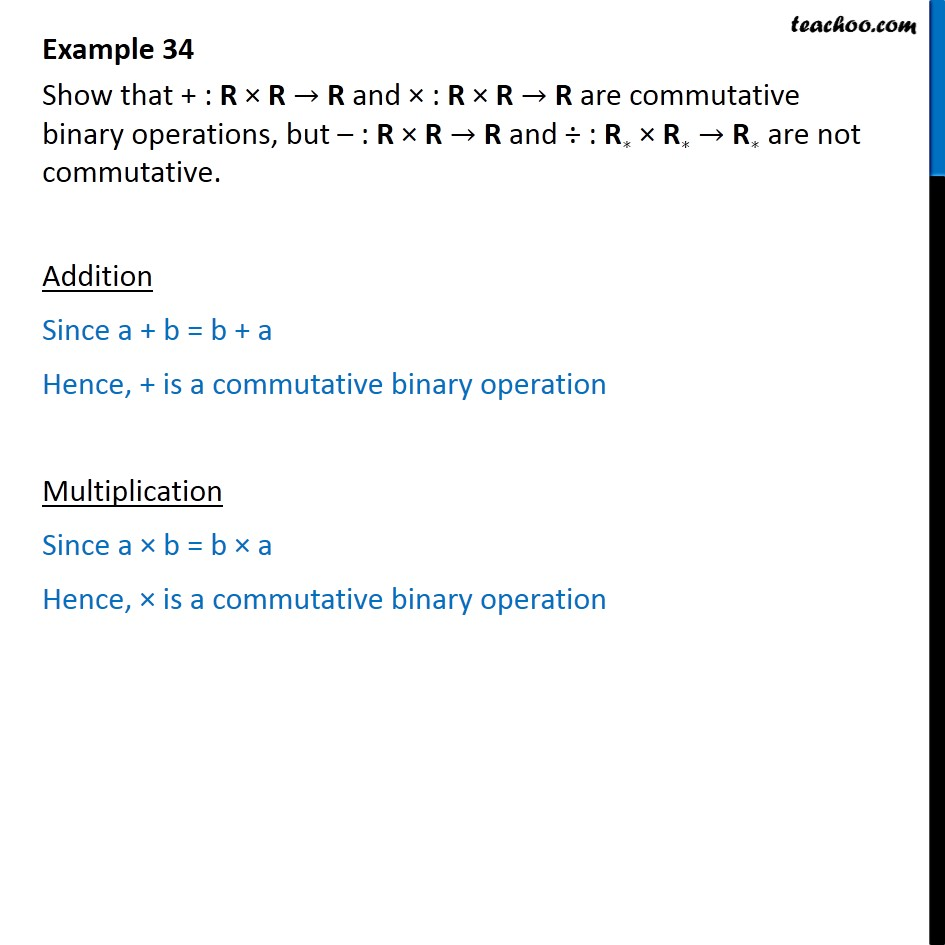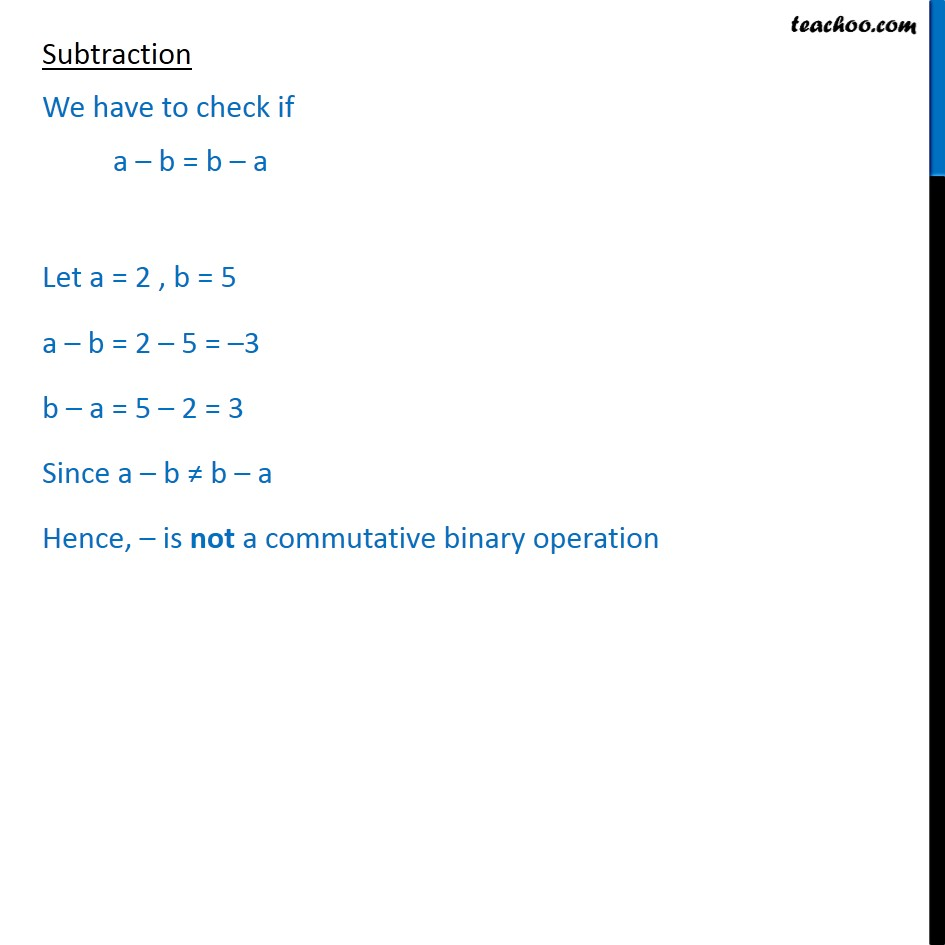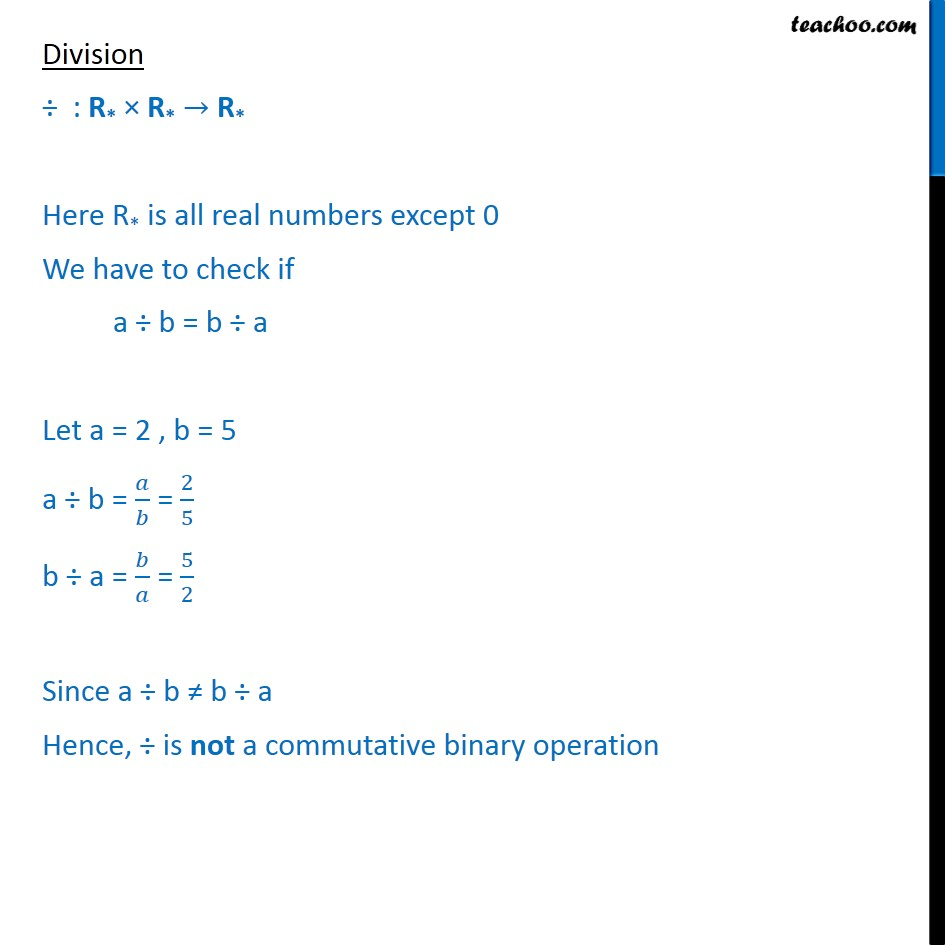Examples

Chapter 1 Class 12 Relation and Functions (Term 1)
Serial order wiseNow learn Economics at Teachoo for Class 12

### Transcript

Example 34 Show that + : R R R and : R R R are commutative binary operations, but : R R R and : R R R are not commutative. Addition Since a + b = b + a Hence, + is a commutative binary operation Multiplication Since a b = b a Hence, is a commutative binary operation Subtraction We have to check if a b = b a Let a = 2 , b = 5 a b = 2 5 = 3 b a = 5 2 = 3 Since a b b a Hence, is not a commutative binary operation Division : R* R* R* Here R* is all real numbers except 0 We have to check if a b = b a Let a = 2 , b = 5 a b = = 2 5 b a = = 5 2 Since a b b a Hence, is not a commutative binary operation